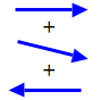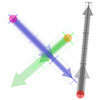#### You may also like### Arrow Arithmetic 1

The first part of an investigation into how to represent numbers using geometric transformations that ultimately leads us to discover numbers not on the number line.### Arrow Arithmetic 2

Introduces the idea of a twizzle to represent number and asks how one can use this representation to add and subtract geometrically.### Arrow Arithmetic 3

How can you use twizzles to multiply and divide?

# Twizzle Twists

##### Age 14 to 16 Challenge Level:

When you draw a large loop with z around its spot, count how many times w turns around its spot.

In Twizzle Wind Up you might have noticed this useful fact:
If your large loop encloses all the z values where w is zero, then the number of times w wound around its spot will tell you how many zeroes there are. This is known as the winding number .

Suppose w winds 3 times around its spot. There will be 3 w -zeroes inside your z -loop, and the formula for w must contain (z-a)(z-b)(z-c) since this is zero when z=a or z=b or z=c . Now all you have to do is to look for a , b , and c inside the loop.